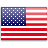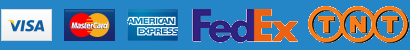Free shipping on all orders over \$ 500

# AbMole Dilution Calculator Calculate the dilution required to prepare a stock solution

The AbMole dilution calculator is based on the following equation:
Concentration(start) x Volume(start) = Concentration(final) x Volume(final)

C1   V1   C2   V2
*   =   *

The AbMole dilution calculator is a useful tool which allows you to calculate how to dilute a stock solution of known concentration.
Enter C1, C2 & V2 to calculate V1.

##### An example of a dilution calculation using the AbMole dilution calculator

What volume of a given 10 mM stock solution is required to make 20ml of a 50 μM solution?

Using the equation C1V1 = C2V2, where C1=10 mM, C2=50 μM, V2=10 ml and V1 is the unknown:

1. Enter 10 into the Concentration (start) box and select the correct unit (millimolar)
2. Enter 50 into the Concentration (final) box and select the correct unit (micromolar)
3. Enter 20 into the Volume (final) box and select the correct unit (milliliter)
4. Press Calculate button
5. The answer of 100 microliter (0.1 ml) appears in the Volume (start) box

##### Resources